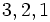# Combinatorics of symmetric group:S3

(diff) ← Older revision | Latest revision (diff) | Newer revision → (diff)
Jump to: navigation, search
This article gives specific information, namely, combinatorics, about a particular group, namely: symmetric group:S3.
View combinatorics of particular groups | View other specific information about symmetric group:S3

This page discusses some of the combinatorics associated with symmetric group:S3, that relies specifically on viewing it as a symmetric group on a finite set.

## Young diagrams and tableaux under the Robinson-Schensted correspondence

### Summary

Partition Number of Young tableaux for that shape Hook length formula Number of permutations (via Robinson-Schensted correspondence) equals square of number of Young tableaux List of permutations (each permutation written using one-line notation)
1 + 1 + 1 1$\frac{3!}{3 \cdot 2 \cdot 1}$ 1$[1,2,3]$
2 + 1 2$\! \frac{3!}{3 \cdot 1 \cdot 1}$ 4$[1,3,2]$,$[3,1,2]$,$[2,1,3]$,$[2,3,1]$
3 1$\! \frac{3!}{3 \cdot 2 \cdot 1}$ 1$[3,2,1]$

Note that the numbers in the first column are also the degrees of irreducible representations, see linear representation theory of symmetric groups and linear representation theory of symmetric group:S3.

### Partition details

Further information: Robinson-Schensted correspondence for symmetric group:S3

Here the partition is the partition for the Young diagram under the Robinson-Schensted correspondence, not the partition for the cycle type of the permutation.

Permutation (one-line notation) Partition (Young diagram) Position tableau Shape tableau$1,2,3$ 1 + 1 + 1$\begin{pmatrix}1 \\ 2 \\ 3 \\\end{pmatrix}$$\begin{pmatrix} 1 \\ 2 \\ 3 \\\end{pmatrix}$$2,1,3$ 2 + 1$\begin{pmatrix}1 & 2 \\ 3 & \\\end{pmatrix}$$\begin{pmatrix}1 & 2 \\ 3 & \\\end{pmatrix}$$1,3,2$ 2 + 1$\begin{pmatrix}1 & 3 \\ 2 & \\\end{pmatrix}$$\begin{pmatrix} 1 & 3 \\ 2 & \\\end{pmatrix}$$2,3,1$ 2 + 1$\begin{pmatrix}1 & 2 \\ 3 & \\\end{pmatrix}$$\begin{pmatrix} 1 & 3 \\ 2 & \\\end{pmatrix}$$3,1,2$ 2 + 1$\begin{pmatrix}1 & 3 \\ 2 & \\\end{pmatrix}$$\begin{pmatrix} 1 & 2 \\ 3 & \\\end{pmatrix}$$3,2,1$ 3$\begin{pmatrix} 1 & 2 & 3 \\\end{pmatrix}$$\begin{pmatrix} 1 & 2 & 3 \\\end{pmatrix}$

## Increase/decrease patterns

One-line notation for permutation Increase/decrease pattern (whether each element is greater or smaller than its predecessor; an I denotes increase, a D denotes decrease Number of Is
1,2,3 II 2
2,1,3 DI 1
1,3,2 ID 1
2,3,1 ID 1
3,1,2 DI 1
3,2,1 DD 0